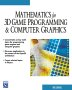# Maths - Matrix Algebra - Determinants

A determinant is a scalar number which is calculated from a matrix. This number can determine whether a set of linear equations are solvable, in other words whether the matrix can be inverted.

## Calculating Determinant

The formula for the determinant is shown here:

## Notation

This scalar number is represented by the matrix with vertical lines on each side: |M|

## Alternative Approaches

Like many mathematical concepts there are are different ways to understand determinants:

### Solving linear equations

If we have n equations with n unknowns then we can solve these equations, provided that these equations are all independent, if they are not, then one equation is derived from another and therefore does not provided any additional information.

### Geometrical Interpretation

Determinants are perhaps most comonly associated with matricies but it does have a geometric interpretaion that is completely independant of matricies (this geometric interpretation is discussed on this page).

#### Independence of vectors (orthogonality)

Perhaps we got a clue to this geometric interpretation when we looked at rotations, pure rotations always have a determinant of one. This is related to the situation where we have a set of unit vectors which are mutually othogonal, the determinant of the matrix formed from these vectors is one.

#### Volume enclosed by vectors

|M| is the volume enclosed by the vectors. However the sign is significant , it may be negative, if an odd number of coordinates are inverted the 'volume' will be negative.

This is related to the tri-vector of a 3D clifford algebra.

### Use to calculate inverse matrix

The formula for calculating the inverse of matrix [M] involve multiplication by the scalar factor 1/|M| so if |M| =0 all the components of the inverse will be infinity indicating, in that case, that [M] does not have an inverse.

## Evaluation of Determinants using Recursion

We can calculate a n×n determinant from a (n-1)×(n-1) matrix and so on until the determinant of a 1×1 matrix which is just the term itself. This recursive method is also known as expansion by minors.

First some terminology, if we remove one row and one column the remaining determinant is known as a minor and the element at the intersection of the rows and columns being removed is known as the cofactor.

 m11 m12 m13 m21 m22 m23 m31 m32 m33

where:

• cofactor
• minor

To evaluate the determinant recursively we first choose any row or column, we then make each term in the row or column into a cofactor.

The determinant is calculated from the sum of minors multiplied by their associated cofactors (alternating the signs) taken over the chosen row or column.

|A| = ∑ aij·Cij

where:

• aij = minor of A (row i and column j removed)
• Cij = cofactor (with sign) = (−1)i+j·mij
• mij = element at row i and column j

#### Example of a 3x3 Matrix

The determinant of:

 m11 m12 m13 m21 m22 m23 m31 m32 m33

is calculated from

first term second term third term
sign + - +
cofactor m11 m12 m13
minor (remove terms with yellow background)
 m11 m12 m13 m21 m22 m23 m31 m32 m33
 m11 m12 m13 m21 m22 m23 m31 m32 m33
 m11 m12 m13 m21 m22 m23 m31 m32 m33

so we get

 m11 m12 m13 m21 m22 m23 m31 m32 m33
= m11
 m22 m23 m32 m33
- m12
 m21 m23 m31 m33
+ m13
 m21 m22 m31 m32

and recursing down to the next level gives:

|M| = m11 m22 m33 + m12 m23 m31 + m13 m21 m32 - m11 m23 m32 - m12 m 21 m33 - m13 m22 m31

## Laplace Expansion

We can either expand along any column (j = 1,2 .. n):

 n det(M) = Σ Mij Cij i=1

or any row (j = 1,2 .. n):

 n det(M) = Σ Mji Cji i=1

where:

• Mij = minor of element ij
• Cji = cofactors of element ij = (-1)i+jMij

## Properties of Determinants

All rotation matrices have determinants of 1

For example |R| = cos(a)2 + sin(a)2 = 1

## Using determinants to solve simultaneous equations

For 3 unknowns

x
-y
z
-1
 m01 m02 v0 m11 m12 v1 m21 m22 v2
 m00 m02 v0 m10 m12 v1 m20 m22 v2
 m00 m01 v0 m10 m11 v1 m20 m21 v2
 m00 m01 m02 m10 m11 m12 m20 m21 m22

## Determinants and Clifford Algebra

 Ax Ay Bx By
= A /\ B = pseudoscalar multiplier

## Calculating Determinant

The formula for the determinant is shown here:

## Code

Very approximate pseudo code for recursive method:

```determinant( A, n )
if n = 2 then return aa - aa
d := 0
for i := 1 to n {
Minor[x][y] = A[x][y] where x != 1 & y != i
d := d + (-1)^(i-1)*a1i*determinant(Minor[i],n-1)
}
return d```

 metadata block see also: Correspondence about this page Book Shop - Further reading. Where I can, I have put links to Amazon for books that are relevant to the subject, click on the appropriate country flag to get more details of the book or to buy it from them.Mathematics for 3D game Programming - Includes introduction to Vectors, Matrices, Transforms and Trigonometry. (But no euler angles or quaternions). Also includes ray tracing and some linear & rotational physics also collision detection (but not collision response). Other Math Books Specific to this page here: Determinant represented by the matrix with vertical lines on each side: |M|

This site may have errors. Don't use for critical systems.